• Sigmoid函数，即f(x)=1/(1+e-x)。神经元的非线性作用函数。（-x是幂数） 人工神经网络的学习算法-BP算法  神经网络的学习是基于一组样本进行的，它包括输入和输出（这里用期望输出表示），输入和输出有多少...

Sigmoid函数，即f(x)=1/(1+e-x)。神经元的非线性作用函数。（-x是幂数）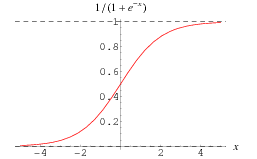人工神经网络的学习算法-BP算法  神经网络的学习是基于一组样本进行的，它包括输入和输出（这里用期望输出表示），输入和输出有多少个分量就有多少个输入和输出神经元与之对应。最初神经网络的权值（Weight）和阈值（Threshold）是任意给定的，学习就是逐渐调整权值和阈值使得网络的实际输出和期望输出一致。  我们假设样本有P个，输入层有N个神经元，隐含层有K个神经元，输出层有M个神经元。Xj为输入层神经元j的输入，Hj为隐含层神经元j的输出，Fj为输出层神经元j的实际输出，Rj为输出层神经元j的期望输出，前一层的输出即为后一层的输入。Whji是输入层神经元i与隐含层神经元j之间的连接权值，Thj是隐含神经元j的阈值，Woji是隐含层神经元i与输出层神经元j之间的连接权值，Toj是输出神经元j的阈值。神经元的非线性作用函数是Sigmoid函数，即f(x)=1/(1+e-x)。from:http://www.shamoxia.com/html/y2009/590.html

顶

3


展开全文• ## OpenGL画点函数

千次阅读 2016-06-11 20:33:18
要描述一个点的几何要素，我们只需要在世界坐标系中指定一个位置，...我们使用下面的OpenGL函数可以指定一个点位置的坐标glVertex *（）；*表示该函数后面有缀码。这些缀码用来指明空间维数 、坐标值变量的数据类型和
要描述一个点的几何要素，我们只需要在世界坐标系中指定一个位置，然后该坐标位置和场景中已有的其他几何描述一起呗传输给观察子程序。除非指定其他属性值，OpenGL图元按默认的大小和颜色来显示。默认的图元是白色的，而默认的点大小等于单一屏幕像素大小。  我们使用下面的OpenGL函数可以指定一个点位置的坐标
glVertex *（）；
*表示该函数后面有缀码。这些缀码用来指明空间维数 、坐标值变量的数据类型和可能的向量形式坐标的描述。
比如：
void drawPointFunc(){
glBegin(GL_POINTS);
glVertex2i(50,100);
glVertex2i(100, 200);
glVertex2i(150, 300);
glEnd();
}
这段代码就是三个在一条线的上的三个孤立点。这些点在2维空间，数据类型是整数类型  后缀码第一位中：代表空间的维数  后缀码第二位中：i(整数)、s(短整数)、f(浮点数)、d(双精度浮点数)  后缀码第三位中：v(“向量”);
下面就是一个根据点画出来的一个三角形
#include "stdafx.h"
#include <GL\glut.h>

void init(){
glClearColor(1.0, 1.0, 1.0, 0.0);
glMatrixMode(GL_PROJECTION);
gluOrtho2D(0.0,200.0,0.0,150.0);
}

void drawLine(){
glClear(GL_COLOR_BUFFER_BIT);
glColor3f(0.0,0.4,0.2);
int point1[] = { 50, 100 };
int point2[] = { 50, 200 };
int point3[] = { 200, 100 };

glBegin(GL_LINES);
glVertex2i(10, 10);
glVertex2i(75, 75);
glEnd();

glBegin(GL_LINES);
glVertex2i(75, 75);
glVertex2i(75, 10);
glEnd();

glBegin(GL_LINES);
glVertex2i(75, 10);
glVertex2i(10, 10);
glEnd();

glFlush();
}

int _tmain(int argc, char** argv)
{
glutInit(&argc,argv);
glutInitDisplayMode(GLUT_RGB | GLUT_SINGLE);
glutInitWindowPosition(100,100);
glutInitWindowSize(400, 400);
glutCreateWindow("画点函数");
init();
glutDisplayFunc(&drawLine);

glutMainLoop();
return 0;
}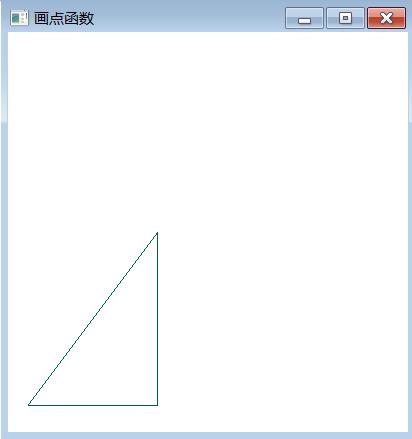赶快自己动手做一下吧！
展开全文opengl
• OpenGL函数 　OpenGL图元默认大小为一个像素单位，默认颜色为白色。函数为： 　glVertex* (); 　星号（*）说明该函数要有后缀码。一般来说，会有2到3个后缀码。第一个后缀码代表维数，二维、三维、四维...
OpenGL画点函数  　　OpenGL图元默认大小为一个像素单位，默认颜色为白色。画点函数为：
glVertex* ();  　　星号（*）说明该函数要有后缀码。一般来说，会有2到3个后缀码。第一个后缀码代表维数，二维、三维、四维坐标分别用2、3、4等数字表示。第二个后缀码代表坐标的数值类型，如i（整数）、s（短整数）、f（浮点）、d（双精度浮点）等。glVertex中可以使用显式的坐标值或引入矩阵形式坐标位置的单个变量，此时需要第三个后缀：v（向量）。
下面例子在斜率为2的直线上绘出了三个等距离的点。
glBegin(GL_POINTS);  　　 glVertex2i (50, 100);  　　 glVertex2i (75, 150);  　　 glVertex2i (100, 200);  　　glEnd();  　　换一种方法，以矩阵形式表示上述坐标值：
int point1 [] = {50, 100};  　　int point2 [] = {75, 150};  　　int point3 [] = {100, 200};  　　glBegin(GL_POINTS);  　　 glVertex2iv (point1);  　　 glVertex2iv (point2);  　　 glVertex2iv (point3);  　　glEnd();  OpenGL画线函数  　　与OpenGL画点函数十分类似，不同在于glBegin()中的符号常量。  　　使用图元常量GL_LINES可连接每一对相邻定点而得到一组直线段。
glBegin(GL_LINES);  　　 glVertex2iv (p1);  　　 glVertex2iv (p2);  　　 glVertex2iv (p3);  　　 glVertex2iv (p4);  　　 glVertex2iv (p5);  　　glEnd();  　　上述代码可以画出线段p1-p2和p3-p4。
使用GL_LINE_STRIP则可以获得折线，如果需要封闭的折线则需要重复某些点坐标。
glBegin(GL_LINE_STRIP);  　　 glVertex2iv (p1);  　　 glVertex2iv (p2);  　　 glVertex2iv (p3);  　　 glVertex2iv (p4);  　　 glVertex2iv (p5);  　　glEnd();  　　上述代码画出折线p1-p2-p3-p4-p5。
生成封闭折线的常量是GL_LINE_LOOP。
glBegin(GL_LINE_LOOP);  　　 glVertex2iv (p1);  　　 glVertex2iv (p2);  　　 glVertex2iv (p3);  　　 glVertex2iv (p4);  　　 glVertex2iv (p5);  　　glEnd();  　　上述代码画出封闭折线p1-p2-p3-p4-p5-p1。
示例代码
include 
展开全文编程 opengl class
• #!/usr/bin/env python # -*- coding: utf-8 -*- # @Time : 18/2/11 上午11:34 ...plt.xlabel('Time(s)') plt.ylabel('Volt') plt.title('PyPlot') plt.xlim(0,10) plt.ylim(-5,5) plt.legend() plt.show()
#!/usr/bin/env python
# -*- coding: utf-8 -*-
# @Time    : 18/2/11 上午11:34
# @Site    :
# @File    : pic.py
# @Software: PyCharm

import  numpy as np
import matplotlib.pyplot as plt

x = np.linspace(-10, 10, 1000)
a = np.sin(x)
b = np.cos(x)
c = np.tan(x)
# d = np.log(x)

plt.figure(figsize=(8,4))
plt.plot(x,a,label='$sin(x)$',color='green',linewidth=0.5)
plt.plot(x,b,label='$cos(x)$',color='red',linewidth=0.5)
plt.plot(x,c,label='$tan(x)$',color='blue',linewidth=0.5)
# plt.plot(x,d,label='$log(x)$',color='grey',linewidth=0.5)

plt.xlabel('Time(s)')
plt.ylabel('Volt')
plt.title('PyPlot')
plt.xlim(0,10)
plt.ylim(-5,5)
plt.legend()
plt.show()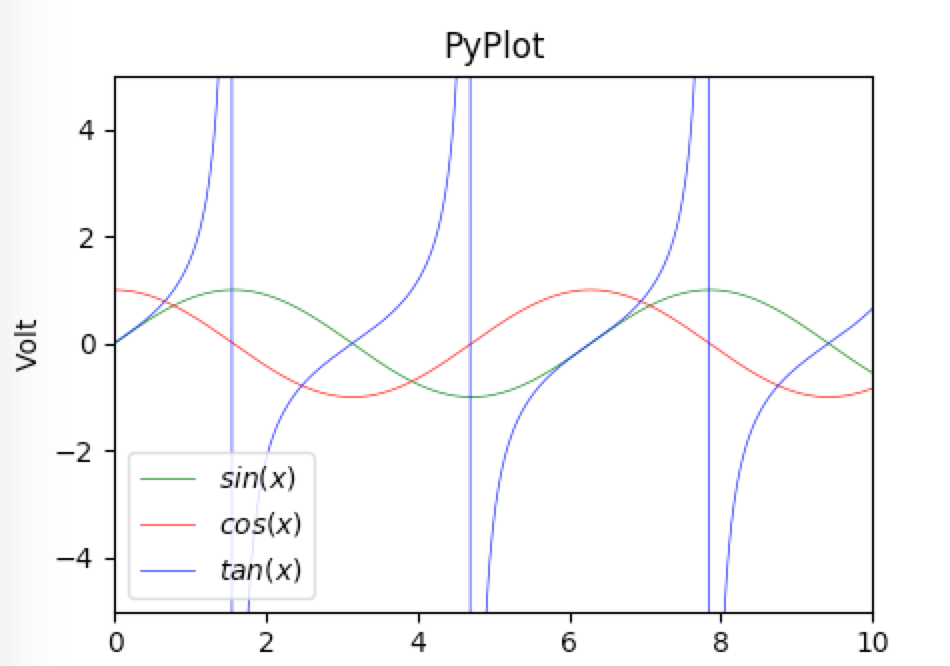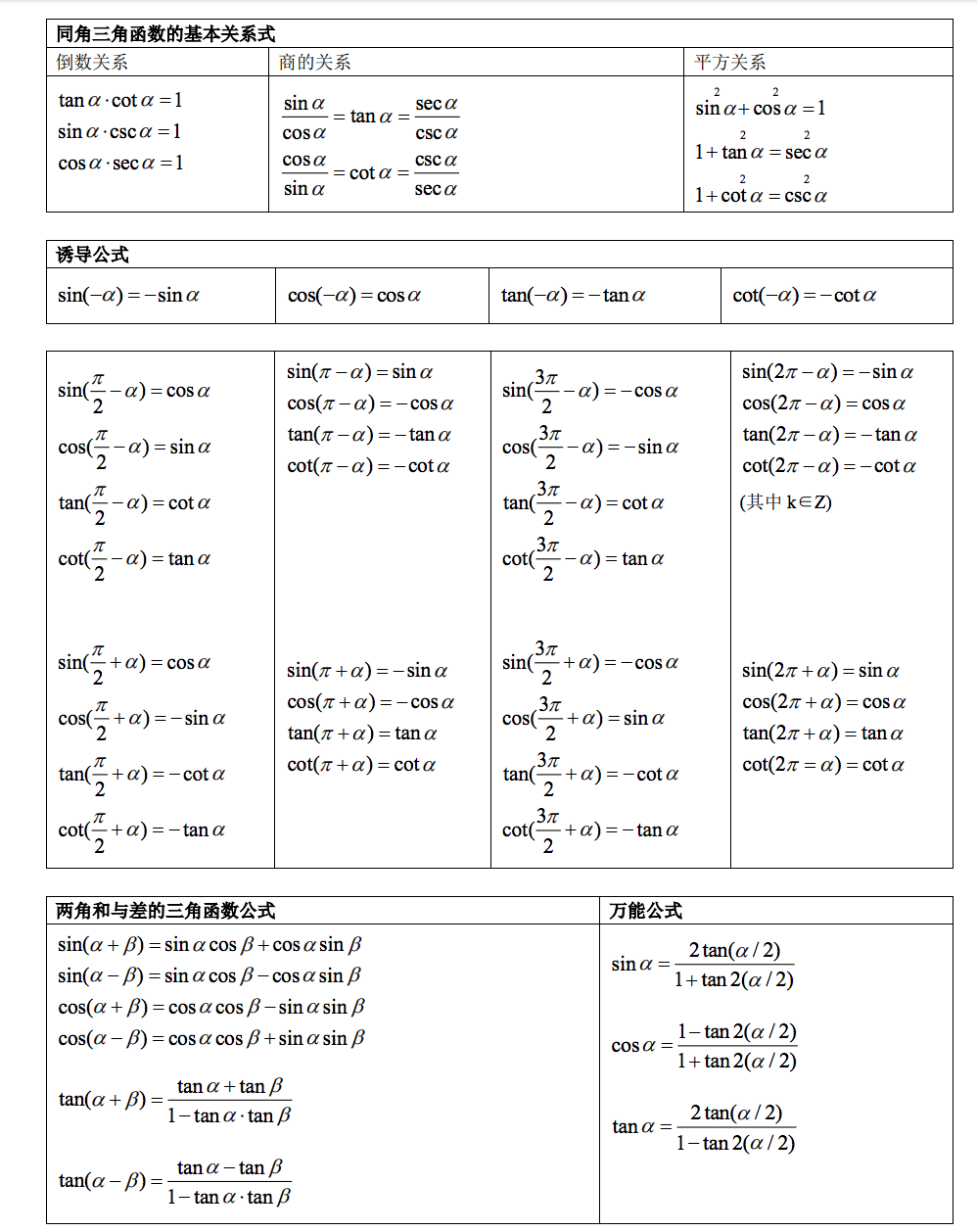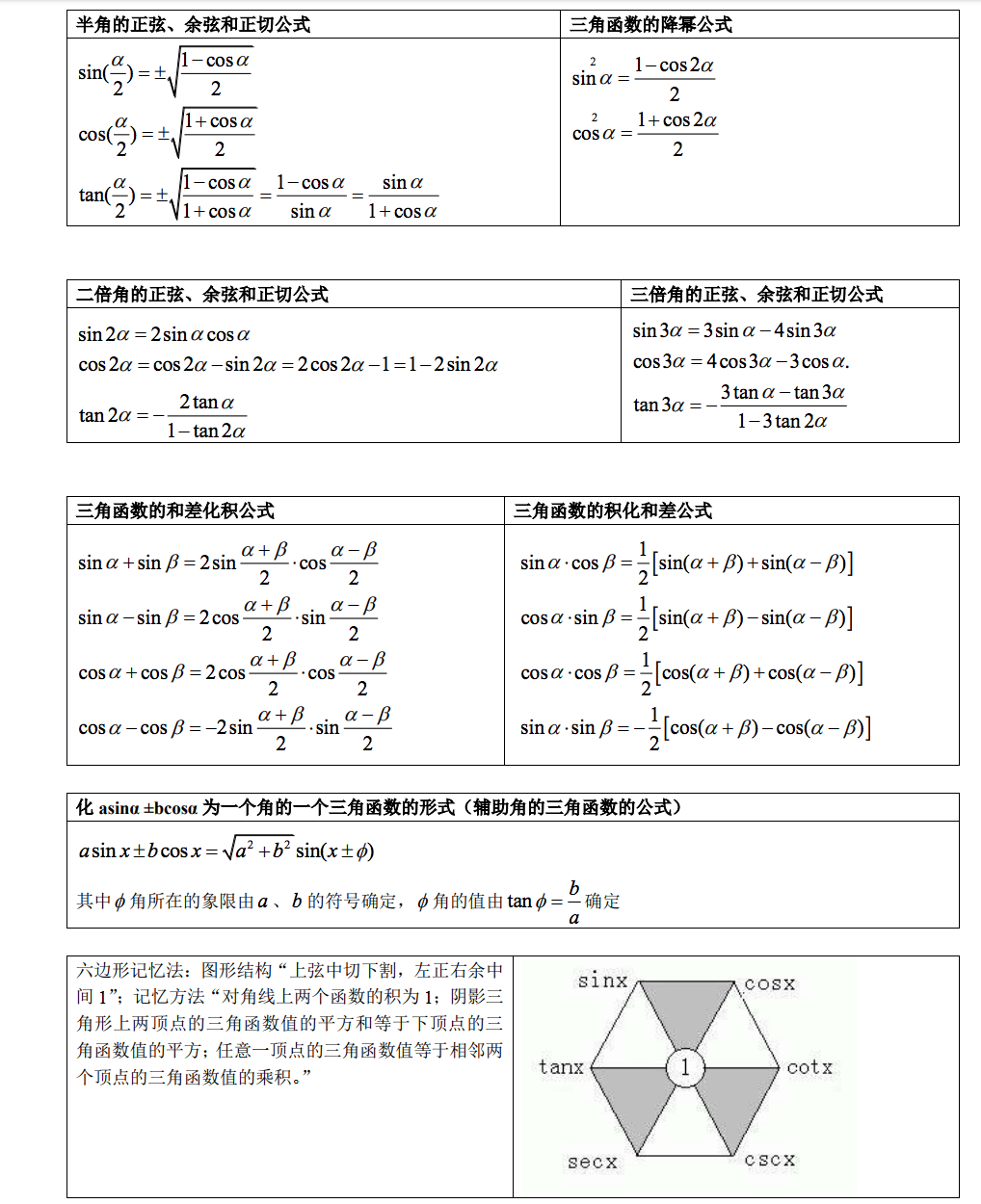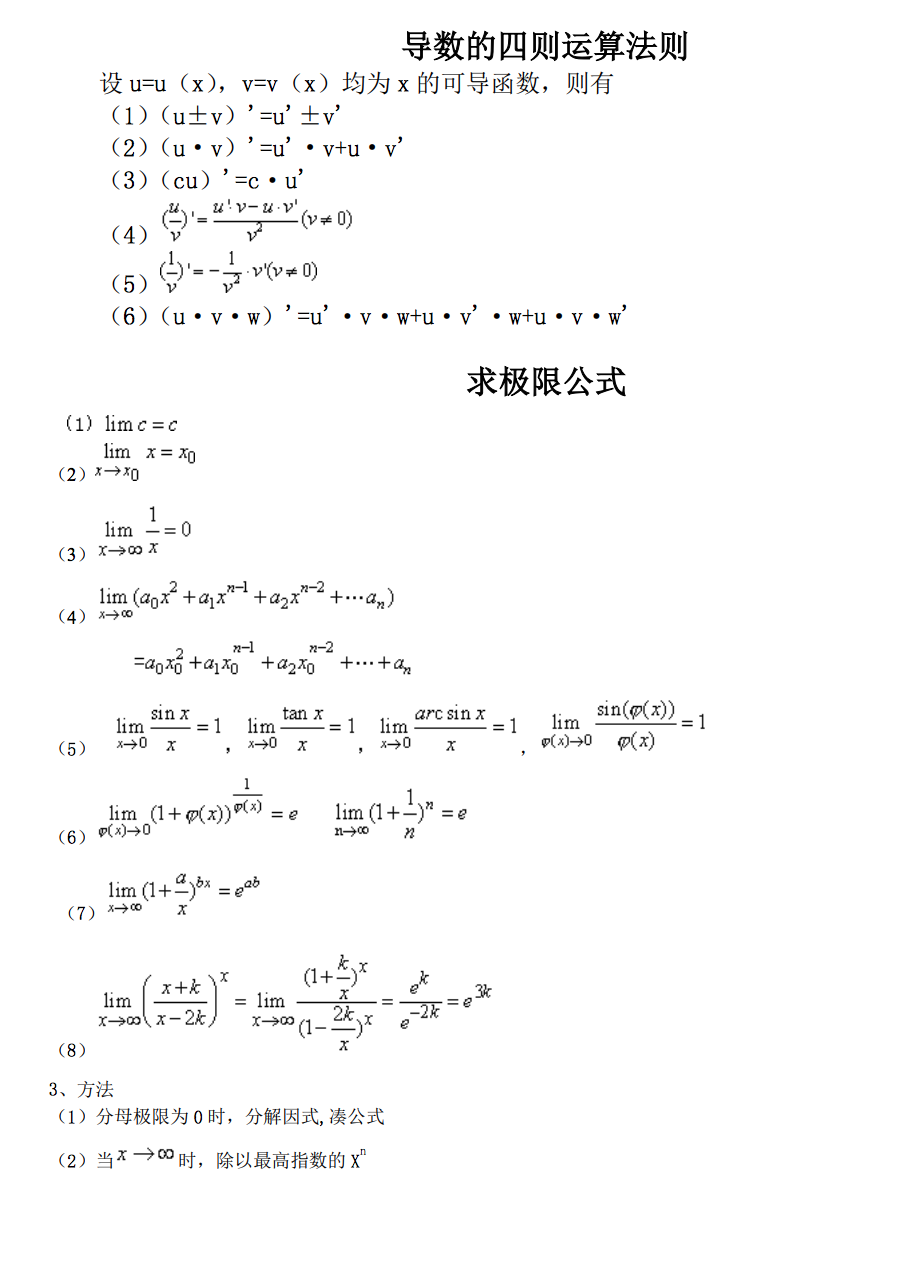展开全文• Matlab中Ksdensity()函数的用途：（概率密度函数） Matlab中Ksdensity()函数的用途：（概率密度函数） http://blog.sina.com.cn/s/blog_6399df820100s8md.html [f,xi] = ksdensity(x) 计算样本向量x的概率密度...
• 原文地址：...第一类贝塞尔函数 在MatLab中用besselj(NU,Z)来表示： 用MatLab的仿真代码是： clear ,clc; format long x=(0:0.01:20)'; y_0=besselj(0,x); y_1
• （一）使用matlab多项式函数图像 在用matlab五次多项式的时候，发现使用y=(0.2771*t^3 - 0.081*t^4 + 0.0063*t^5);总是会报错。搜了搜资料，发现可以这么： 例如：五次多项式（quintic polynomial） y=0.2771...python matlab 数学建模
• pt.text(0.0,-0.5,'don`s move',fontdict={'size':'16','color':'b'}) # 添加标注，xy是标注箭头要指的点，xytext：描述信息的坐标，arrowprops箭头的样式 pt.annotate('note!!',xy=(-np.pi/2.0,-1),xytext=(-np.pi/...
• 转自:http://blog.sina.com.cn/s/blog_9bf272cf01015i9b.html UIView *singleMapView = [[UIView alloc] initWithFrame:CGRectMake(10, 150, 300, 250)]; singleMapView.backgrou
• 使用MATLAB进行传递函数S域Z域的相互转换，且绘制S域Z域的伯德图
• 缓动函数包括quad、cubic、sine、quint、circt、bounce、back、expo、elastic、quart等等 转载自：https://www.jianshu.com/p/c157c5898153 Zszen 图示 下图所示，所有公式的作用域都是从0～1 简介 ...Tween ease
• 用参数zorder调节，zorder整数越大，显示时越靠上。 举个栗子： plt.plot(a, b, color='r', marker='o', zorder=1) plt.scatter(x, y, marker='x', color='r', s=50, zorder=2) 成功。Python
• OpenGL函数  要描述一个点的几何要素，我们只需在世界坐标系中指定一个位置。然后该坐标位置和场景中 已有的其他几何描述一起传递给观察函数。除非指定其他属性值， OpenGL图元按默认的大小和颜 色来显示 。...计算机图形学
• 常见的线型字符有：'-'(直线), '--'(虚线), ':'(点线), '-.'(点线) 等。常见的颜色字符有：'r'(red,红色), 'b'(blue，蓝色), 'g'(green,绿色), 'c'(cyan,青色), 'k'(black,黑色), 'w'(white,白色), 'm'(magenta,...
• 函数：f(t)=e(−0.1+jπ2)tf(t)=e^{(-0.1+j\frac {\pi }{2})t}f(t)=e(−0.1+j2π​)t 代码： clc clear s=-0.1+1j*pi/2; t=0:0.01:30; f=exp(s.*t); x=t; y=imag(f); z=real(f); plot3(x,y,z,'-black'); grid on ...matlab 三维图
• 在学深度学习的时候，会遇到激活函数，下面就用matlab出sigmoid和tanh的函数图像，直观感受一下。x=linspace(-10.0,10.0); y=1./(1.0+exp(-1.0*x)); plot(x,y)这里linspace是matlab中的指令，用来产生线段中间的N...
• sigmod(x)函数sigmod函数的数学公式为: Θ(x)=11+e−x \Theta(x)=\frac {1}{1+e^{-x} } 函数取值范围(0,1),函数图像下图所示:二. tanh(x) 函数tanh(x)函数的数学公式为：tanh(x)=sinh(x)cosh(x)tanh(x) = \frac{...
• 传递函数的极点就是对应微分方程的特征根（回忆一下，$\frac{1}{s+a}$是$e^{-a t}$的Laplace变换），因此它们决定了系统自由运动的模态。 传递函数的零点不直接形成自由运动的模态，但会影响各模态在响应中的比重。...
• 一、函数的返回值可以通过人return 来指定函数的返回值用一个变量来接收返回值return 后面跟什么值，函数就会返回什么值# 在函数中return后的代码都不会被执行，return一旦执行，函数自定结束例如： print('jaaj')...
• MATLAB中plot函数常常被用于绘制各种二维图像，其用法也是多种多样，本文仅介绍plot函数的基本用法——使用plot函数绘制二维点图和线图。plot函数的一般调用形式如下： plot(X, Y, LineSpec) 其中X由所有输入点坐标...matlab plot
• matplotlib 基础练习:一元二次函数 样例： 1、安装matplotlib模块 Matplotlib 是 Python 的绘图库。 安装方法： pip install matplotlib -i ...python numpy matlab
• [问题:]直接求直线于弧线(圆角矩形的弧线部分)的交点的函数,我觉得应该是没有的. [问题背景:]在绘画进度条控件中, 进度滑块当处于一开始和快完成,有弧度部分的时候,想要看的过程比较自然,就要自己手动填充这一部分...drawPie
• MATLAB里面自带了K线的函数candle自带函数效果预览图原始candle的使用方法语法描述输入参数修改源代码原始代码修改部分最终显示预览修改后的完整代码 自带函数效果预览图 可以看出K线图有基本的框架，但是和...matlab 股票 candle
• transition-timing-function属性指的是过渡的“缓动函数”。主要用来指定浏览器的过渡速度，以及过渡期间的操作进展情况，其中要包括以下几种函数： (单击图片可放大) 案例展示： 在hover状态下，让...
• if isinstance(writer, six.string_types): if writer in writers.avail: writer = writers[writer](fps, codec, bitrate, extra_args=extra_args, ... "MovieWriter %s unavailable" % writer)python...